and related topics

Balmoral Software

Solutions: 4

### Problem Definition

The first part of this paper discusses cyclic Heronian quadrilaterals (CHQs), four-sided convex polygons that have integer sides and area, and can be inscribed in a circle. Cyclic quadrilaterals have the maximum area amongst all quadrilaterals having the same sides [A]. For example, a square has greater area than any rhombus with the same side length. Equable non-cyclic Heronian quadrilaterals, such as certain ones created from three congruent right triangles, are discussed in a later section.

In general, we will consider cases where the area A of a CHQ is a rational factor t times its perimeter P:

 A = tP, 1 ≤ t ≤ 3, 
so that t = 1 corresponds to the equable case. If t > 1, it is assumed to be of the form t = h/(h - 2) for some integer h ≥ 3, and will be used to determine equable quadrilateral prisms.

Exactly four CHQs are equable:

Sides (in any order)Perimeter = Area
(4,4,4,4)16
(6,6,3,3)18
(8,5,5,2)20
(14,6,5,5)30
Following is a proof of the results for the general relation  that includes the four equable quadrilaterals as a subcase.

Let the positive integer sides of a CHQ be a, b, c, d, and denote by s its semiperimeterBy the generalized polygon inequality, the sum of any three sides of a non-degenerate quadrilateral must exceed the fourth, so
 a < b + c + d, 
and s - a, s - b, s - c and s - d are all strictly positive. Using Brahmagupta's formula for the area of a cyclic quadrilateral, we have
 A2 = (s - a)(s - b)(s - c)(s - d) 
If the perimeter P = 2s = a + b + c + d is odd, then s is of the form k + 1/2 for some positive integer k, and so is each of the four factors in A2, whence the product is of the form m + n/16 for positive integers m and n, n < 16. Therefore, the area and the sides cannot all be integers if P is odd. Henceforth, we will assume that the perimeter is even so that the area is an integer. It follows that the semiperimeter s is also an integer.

The cyclic area formula  does not distinguish between different orderings of the sides, so without loss of generality, we can assume that the integer sides of a CHQ are ranked alphabetically from longest to shortest:

 a ≥ b ≥ c ≥ d ≥ 1 

### Change of variables

To simplify the algebra, we introduce new integers w, x, y, and z:
w = s - a

x = s - b

y = s - c

z = s - d

These integers are all strictly positive by the generalized polygon inequality. Summing both sides of the equations above, we have
w + x + y + z = 4s - (a + b + c + d) = 4s - 2s = P
The square of the CHQ area can now be written more simply as
A2 = wxyz
The inverse relationships between w, x, y, z and the sides of the quadrilateral are:
a = s - w

b = s - x

c = s - y

d = s - z

From  and , we have
1 ≤ d ≤ c ≤ b ≤ a < b + c + d
which can be written in terms of the new variables as
 1 ≤ w ≤ x ≤ y ≤ z ≤ w + x + y - 2 
This is the fundamental relationship between w, x, y and z that will form the foundation for the analysis below. Variables in the relationship above are unbounded, so to find all CHQs satisfying , we will determine limits for w, x and y. All solutions can then be found by a computer-aided search over a finite and manageable set of possibilities.

We begin by establishing a general technique that will be useful in the following sections. Consider a differentiable real-valued multivariate function f(x1,x2,...,xn) over the open domain of nested real variables

 x1 ≤ x2 ≤ ... ≤ xn 
Assume the following three conditions hold:

Condition 1: The partial derivatives are nested in reverse order over the domain :

 ∂f/∂xn ≤ ∂f/∂xn-1 ≤ ... ≤ ∂f/∂x1 
Condition 2: There exists a lower bound L such that
 f(L,L,..,L) > 0 
Condition 3: The partial derivative
 ∂f/∂dxn > 0 for x1 ≥ L 
Then it follows that
f(x1,x2,...,xn) > 0
over the domain L ≤ x1 ≤ x2 ≤ ... ≤ xn.

Proof. By ,  and , f is strictly increasing in all variables when x1 ≥ L. The additional condition  establishes the conclusion.

Define the multivariate function Ft as follows:

Ft(w,x,y,z) = A2 - t2P2 = wxyz - t2(w + x + y + z)2,

1 ≤ w ≤ x ≤ y ≤ z ≤ w + x + y - 2, 1 ≤ t ≤ 3,

where t is the multiplicative factor defined in . From the given constraints
 w ≤ x ≤ y ≤ z, 
we have
1/z ≤ 1/y ≤ 1/x ≤ 1/w

wxy - 2t2(w + x + y + z) ≤ wxz - 2t2(w + x + y + z) ≤ wyz - 2t2(w + x + y + z) ≤ xyz - 2t2(w + x + y + z)

dFt/dz ≤ dFt/dy ≤ dFt/dx ≤ dFt/dw

for all t and all values of w, x, y and z in the domain , which establishes Condition 1 for the function Ft.

### Limiting the values of w

Consider the minimum value of w for which Ft(w,w,w,w) is positive:
 Ft(w,w,w,w) = w4 - t2(4w)2 > 0 w > 4t, 
which holds for all t if
w ≥ 13,
so that this lower bound establishes Condition 2 for the function Ft. We have
z ≤ w + x + y - 2 < w + x + y

wxy - 2t2(w + x + y + z) > wxy - 4t2(w + x + y),

so
dFt/dz > Gt(w,x,y)
for
Gt(w,x,y) = wxy - 4t2(w + x + y).
From the given constraints ,
1/y ≤ 1/x ≤ 1/w

wx - 4t2 ≤ wy - 4t2 ≤ xy - 4t2

dGt/dy ≤ dGt/dx ≤ dGt/dw

for all t and all values of w, x and y in the domain , which establishes Condition 1 for the function Gt. Consider the minimum value of w for which Gt(w,w,w) > 0:
Gt(w,w,w) = w3 - 4t2(3w) > 0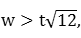which is already satisfied by . Therefore, Condition 2 is established for the function Gt. From  and , we can write
wx ≥ w2 > (4t)2 > 4t2

dGt/dy = wx - 4t2 > 0,

which establishes Condition 3 for Gt, and we conclude that Gt(w,x,y) > 0 over the domain 13 ≤ w ≤ x ≤ y. Therefore dFt/dz > 0, which establishes Condition 3 for the function Ft, and it follows that Ft is strictly positive when w ≥ 13. Summarizing, we have the following table:
wxwxySolutions to A = tP
w ≥ 13anyanyanynone (Ft > 0 for all t)
1 ≤ w ≤ 12anyanyanyTBD

### Limiting the values of x

Now assume 1 ≤ w ≤ 12, and consider Ft to be a function of x, y and z that is parametric in the finite number of integer values for w. Condition 1 still holds for Ft on the domain . Applying the extreme values of w and t, we have
Ft(w,x,x,x) = wx3 - t2(3x + w)2 ≥ (1)x3 - (3)2(3x + 12)2 > 0

x3 - 81(x + 4)2 > 0

The solution to this cubic inequality is x > 88.4885, or
 x ≥ 89, 
which establishes Condition 2 for the function Ft. As in the preceding section, Condition 3 for Ft is established when
Gt(w,89,89) > 0
and
dGt/dy > 0
for all w. Condition 1 still holds for Gt on the domain . Again applying the extreme values of w and t, the former inequality is
w(89)2 - 4t2[w + 2(89)] ≥ (1)(89)2 - 4(3)2[12 + 2(89)] = 1081 > 0,
which establishes Condition 2 for Gt. From , we can write
x ≥ 89 > 36 = 4(3)2 ≥ 4t2 ≥ 4t2/w

wx > 4t2

dGt/dy = wx - 4t2 > 0,

which establishes Condition 3 for Gt and Ft, and it follows that Ft is strictly positive when 1 ≤ w ≤ 12 and x ≥ 89:
wxwxySolutions to A = tP
w ≥ 13anyanyanynone (Ft > 0 for all t)
1 ≤ w ≤ 12x ≥ 89anyanynone (Ft > 0 for all t)
1 ≤ w ≤ 12w ≤ x ≤ 88anyanyTBD

### Limiting the values of wx

Applying the extreme values of z, we have
Ft(w,x,y,z) = wxyz - t2(w + x + y + z)2

< wxy(y + w + x) - t2(2y + w + x)2

= (wx - 4t2)y2 + (wx - 4t2)(w + x)y - t2(w + x)2

If wx - 4t2 ≤ 0, then all coefficients of this quadratic in y are zero or negative, and Ft < 0 for all values of y. Based on this result, the last case in the preceding table can be subdivided as follows:
wxwxySolutions to A = tP
w ≥ 13anyanyanynone (Ft > 0 for all t)
1 ≤ w ≤ 12x ≥ 89anyanynone (Ft > 0 for all t)
1 ≤ w ≤ 12w ≤ x ≤ 88wx ≤ 4t2anynone (Ft < 0 for all t)
1 ≤ w ≤ 12w ≤ x ≤ 88wx > 4t2anyTBD

### Limiting the values of y

Next, we assume that 1 ≤ w ≤ 12, w ≤ x ≤ 88 and wx > 4t2. If 4t2 ∈ ℤ, then wx ≥ 4t2 + 1, but if 4t2 ∉ ℤ, then
wx ≥ ⌈4t2⌉ = ⌊4t2⌋ + 1,
where ⌈⌉ and ⌊⌋ are the ceiling and floor functions, respectively. Thus, in all cases,
 wx ≥ ⌊4t2⌋ + 1 
From the prism convention in the Introduction, we have
1/289 ≤ ⌊4t2⌋ - 4t2 + 1 ≤ 1,
or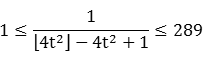A minimum for A2 is
A2 = (wx)yz ≥ (⌊4t2⌋ + 1)y2
by , and a maximum for t2P2 is
t2P2 = t2(w + x + y + z)2

≤ t2(w + x + y + w + x + y - 2)2

≤ t2[2(12) + 2(88) + 2y - 2]2

= 4t2y2 + 792t2y + 39,204t2

Therefore, A2 > t2P2 will occur when the minimum value of A2 exceeds the maximum value of t2P2:
(⌊4t2⌋ + 1)y2 > 4t2y2 + 792t2y + 39,204t2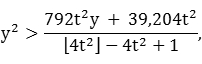which holds if
y2 > 289(792y + 39,204)t2,
which in turn holds for all t if
y2 - 2,059,992y - 101,969,604 > 0,
The solution to this quadratic inequality isor
y ≥ 2,060,042
(Tighter bounds on y can be found by considering individual cases for values of w.)

Based on this result, the last case in the preceding table can be subdivided as follows:

wxwxySolutions to A = tP
w ≥ 13anyanyanynone (Ft > 0 for all t)
1 ≤ w ≤ 12x ≥ 89anyanynone (Ft > 0 for all t)
1 ≤ w ≤ 12w ≤ x ≤ 88wx ≤ 4t2anynone (Ft < 0 for all t)
1 ≤ w ≤ 12w ≤ x ≤ 88wx > 4t2y ≥ 2,060,042none (Ft > 0 for all t)
1 ≤ w ≤ 12w ≤ x ≤ 88wx > 4t2x ≤ y ≤ 2,060,041TBD

### Enumeration of solutions

Now that we have found finite bounds for all variables w, x, y and z, searching for CHQs with the property that Ft = 0 is a manageable problem that can be solved on a computer in a few minutes. At the expense of finding and discarding a relatively few extraneous results when wx ≤ 4t2, we can ignore the restriction on wx and focus solely on the finite bounds for w, x, y and z. The number of outcomes to be considered then is: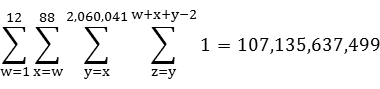For each of these possibilities, the following steps will be completed:
Verify that P = w + x + y + z is even
Verify that A2 = wxyz is a square
If A = P, output results for equable quadrilaterals
If A > P and 2A/(A - P) is an integer, output results for equable quadrilateral prisms
If A < P and wx > 4, output preliminary results for perimeter-dominant CHQs
The four equable CHQs are listed below, in order of increasing area:
abcdA=PwxyzPossible shapes (depending on order of sides)
4444164444Square
6633183366Rectangle, right kite
8552202558Isosceles trapezoid, trapezium*
1465530191010Isosceles trapezoid, trapezium*

*: American definition
The first three of these are bicentric since each has the square of its area equal to the product of its side lengths (A2 = abcd).

### Plots

This section presents a method for creating scale drawings of cyclic quadrilaterals and their circumcircles. Along with the side lengths and the sequence in which they appear, one of the two diagonals of a convex quadrilateral is needed to fix its interior angles and area. For consistent display purposes, we will follow the convention of orienting a quadrilateral with its longest side a along the horizontal x-axis and the origin at its left endpoint. The other sides b,c,d are arranged clockwise around the quadrilateral in decreasing order, and the quadrilateral inequality
a < b + c + d
must be satisfied. The general form of the quadrilateral then is: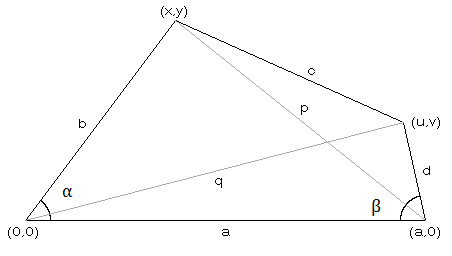where y and v are positive. Let p be the length of the descending diagonal joining points (x,y) and (a,0), and let q be the length of the ascending diagonal joining the origin to the point (u,v). By Ptolemy's Theorem, formulas for the diagonals are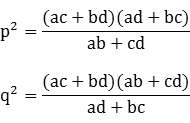If α is the angle between sides a and b, then by the Law of Cosines, we have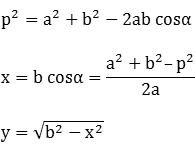Similarly, if β is the angle between sides a and d, then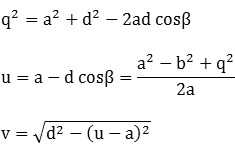The circumcircle of the quadrilateral passes through the non-collinear points (0,0), (a,0) and (u,v). As shown in the results for equable triangles, the center and radius of the circle defined by these three points are: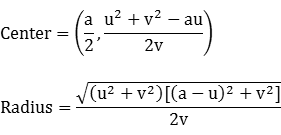Evaluating the formulas for the vertex coordinates and circumcircle parameters of each equable CHQ, we have the following table:
44443232(4,0)(0,4)(4,4)(2,2)
6633144/545(6,0)(18/5,24/5)(6,3)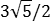(3,3/2)
8552412500/41(8,0)(3,4)(310/41,80/41)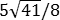(4,1/8)
1465510000/109109(14,0)(546/109,360/109)(10,3)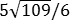(7,-31/6)
The results above can be used to create scale drawings of the four equable CHQs and their corresponding circumcircles: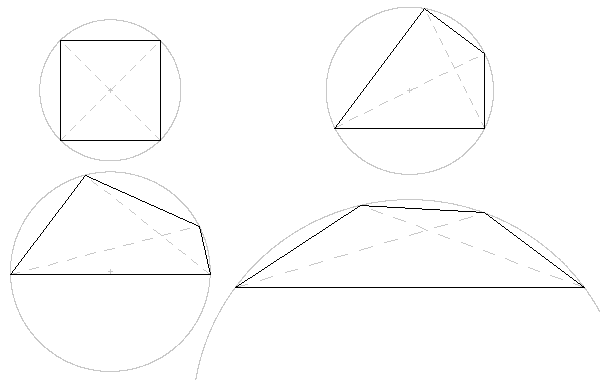The first 14 perimeter-dominant CHQs were found using the search algorithm in a preceding section, subject to the constraint wx > 4, and are listed here in order of increasing perimeter:
abcdPAP-Awxyz
333312933333
4433141223344
5533161513355
7551181622448
9551201551559
8741201822369
10552221841669
117422420415810
11553242131779
12554262421889
13555282711999
148713028217814
15106132302161015
20165142402151620
From the table in a preceding section, all other solutions for perimeter greater than integer area (F1 < 0) occur when wx ≤ 4. Since we also have x ≥ w, this results in exactly five different value pairs for (w,x):
(w,x) ∈ {(1,1),(1,2),(1,3),(1,4),(2,2)},
but the value of y is unbounded. Define the integer k as
k = z - y, where 0 ≤ k ≤ w + x - 2 by 
The perimeter can then be written
P = 2y + w + x + k,
and must be even in order for the quadrilateral area to be an integer, so k and w + x - 2 have the same parity. We then have the following cases where the perimeter may exceed the integer area:
Case(w,x)w + x - 2k
A(1,1)00
B(1,2)11
C(1,3)20,2
D(1,4)31,3
E(2,2)20,2
Each subcase can be denoted by the capital letter for the main case followed by a feasible value for k; for example, Case C2 occurs when (w,x) = (1,3) and k = 2. In each subcase, the squared area is wxy(y + k), which may have infinitely-many solutions for y. Some of these infinite sequences of solutions for y have been found in the Online Encyclopedia of Integer Sequences (oeis.org), as shown below:

Case A0: (w,x,y,z) = (1,1,y,y)

A2 = y2 is a square for all values of y

P = 2y + 2 = 2A + 2 is a line in the P-A plane

Examples:
wxyzPAP-Aabcd
11114131111
11226242211
11338353311
114410464411
115512575511
116614686611
...

Case B1: (w,x,y,z) = (1,2,y,y+1)

 w x c d y z P A P-A a b 1 2 8 9 20 12 8 9 8 2 1 1 2 49 50 102 70 32 50 49 2 1 1 2 288 289 580 408 172 289 288 2 1 1 2 1681 1682 3366 2378 988 1682 1681 2 1 1 2 9800 9801 19604 13860 5744 9801 9800 2 1 1 2 57121 57122 114246 80782 33464 57122 57121 2 1 1 2 332928 332929 665860 470832 195028 332929 332928 2 1 1 2 1940449 1940450 3880902 2744210 1136692 1940450 1940449 2 1 1 2 11309768 11309769 22619540 15994428 6625112 11309769 11309768 2 1 ... OEIS: A001108 A001542 A055997
The perimeter and area formulas are
P = 2y + 4

A2 = 2y(y + 1)

(P - 3)2 - 2A2 = 1,

which is a modified Pell equation with parameters D = 2 and p = 3. The associated coefficients are a1 = 1, b1 = -3, a2 = 1, b2 = 0, so 2b1(p - 1)/a1 = -12, 2b2(p - 1)/a2 = 0, and recursive solutions for the perimeter and area are:
Pn = 6Pn-1 - Pn-2 - 12, P0 = 20, P1 = 102

An = 6An-1 - An-2, A0 = 12, A1 = 70

Case C0: (w,x,y,z) = (1,3,y,y)
A2 = 3y2 has no solution in integers
Case C2: (w,x,y,z) = (1,3,y,y+2)
 w x c d y z P A P-A a b 1 3 6 8 18 12 6 8 6 3 1 1 3 25 27 56 45 11 27 25 3 1 1 3 96 98 198 168 30 98 96 3 1 1 3 361 363 728 627 101 363 361 3 1 1 3 1350 1352 2706 2340 366 1352 1350 3 1 1 3 5041 5043 10088 8733 1355 5043 5041 3 1 1 3 18816 18818 37638 32592 5046 18818 18816 3 1 1 3 70225 70227 140456 121635 18821 70227 70225 3 1 1 3 262086 262088 524178 453948 70230 262088 262086 3 1 ... OEIS: A092184 A102206 A005320 A102206 A092184
The perimeter and area formulas are
P = 2y + 6

A2 = 3y(y + 2)

(P/2 - 2)2 - 3(A/3)2 = 1

Since P is even, P/2 - 2 is an integer. The product 3y(y + 2) mod 9 is either 0 or 6 and a square mod 9 is one of {0,1,4,7}. The only common remainder is A2 mod 9 = 0, so A/3 is an integer and the preceding is a modified Pell equation with parameters D = 3 and p = 2. The associated coefficients are a1 = 1/2, b1 = -2, a2 = 1/3, b2 = 0, so 2b1(p - 1)/a1 = -8, 2b2(p - 1)/a2 = 0, and recursive solutions for the perimeter and area are:
Pn = 4Pn-1 - Pn-2 - 8, P0 = 18, P1 = 56

An = 4An-1 - An-2, A0 = 12, A1 = 45

Case D1: (w,x,y,z) = (1,4,y,y+1)
A2 = 4y(y + 1) = (2y + 1)2 - 1

(2y + 1)2 - A2 = 1

has no solution in integers.

Case D3: (w,x,y,z) = (1,4,y,y+3)

A2 = 4y(y + 3) = (2y + 3)2 - 9

(2y + 3)2 - A2 = 9

cannot occur for y ≥ x = 4 (or equivalently, 2y + 3 ≥ 11) since the next lower square will be at least 21 less.

Case E0: (w,x,y,z) = (2,2,y,y)

A2 = 4y2 is a square for all values of y

P = 2y + 4 = A + 4 is a line in the P-A plane

Examples:
wxyzPAP-Aabcd
22228442222
223310643322
224412844422
2255141045522
2266161246622
2277181447722
...
There are exactly two instances where Case E0 produces the same perimeter and integer area as found in the preceding table of results for wx ≥ 5. These duplicates are:
(w,x,y,z) = (2,2,9,9) or (1,6,6,9), P = 22 & A = 18
(w,x,y,z) = (2,2,10,10) or (1,5,8,10), P = 24 & A = 20
Case E2: (w,x,y,z) = (2,2,y,y+2)
A2 = 4y(y + 2) = (2y + 2)2 - 4

(2y + 2)2 - A2 = 4

has no solution in integers since the difference of two positive squares cannot be 4.

A scatter diagram shows all the perimeter-dominant CHQs in the P-A plane, with each (P,A) pair represented by a pixel. In this diagram, the perimeter P increases from left to right and the area A from bottom to top, so perimeter-dominant solutions are in the lower right portion of the diagram. For reference, the points P = A are shown as a dimmed diagonal line. A zoomed-in version shows more detail for smaller values of P and A.

The 4 equable cases are shown in purple on the scatter diagrams, and the 14 initial perimeter-dominant cases in the table above are shown in black. Other colors are allocated according to the following summary table:

 Case y-sequence A P Diagram color A0 Any positive integer y 2y + 2 Blue B1 8,49,288,1681,9800,...(OEIS A001108)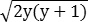(OEIS A001542) 2y + 4 Orange C2 6,25,96,361,1350,...(OEIS A092184)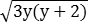(OEIS A005320) 2y + 6 Green E0 Any integer ≥ 2 2y 2y + 4 Red(except for twoduplicates mentioned above)

### Minimum area of a convex quadrilateral

Unlike a triangle, which is rigidly fixed by its side lengths, a convex quadrilateral with sides specified in length and order can have a range of areas, depending on its interior angles. In essence, the quadrilateral can "flex" unless a diagonal connecting its opposite vertices is also specified, at which point the quadrilateral becomes fixed in orientation and area.

The minimum area of a convex quadrilateral with specified lengths and order of sides can be determined from the areas of the six triangles formed by combining two of the quadrilateral sides into a straight line segment [B] [C]. To allow a zero minimum area for convex quadrilaterals that can be completely collapsed (such as any rhombus), a non-strict triangle inequality applies:

 max{x,y,z} ≤ (x + y + z)/2 
for a triangle with sides x,y,z. The minimum area of a convex quadrilateral with sides {a,b,c,d} (in any order) is
min{T(a+b,c,d),T(a+c,b,d),T(a+d,b,c),T(b+c,a,d),T(b+d,a,c),T(c+d,a,b)}
where T(x,y,z) represents the area of triangle {x,y,z}. A triangular area term is excluded from consideration if  is not satisfied. For example, the convex quadrilateral {7,3,4,5} has associated triangle areas
T(7,7,5) = 16.346
T(8,7,4) = 13.998
T(9,7,3) = 8.786
so its minimum area is 8.786. Clearly, all minimum-area convex quadrilaterals are non-cyclic since three of their vertices are collinear and therefore cannot be on a circumcircle.

The minimum and maximum areas of the 6,328 integer-sided quadrilaterals with perimeters up to 50 are listed here. The order of sides is arbitrary since all arrangements are considered for the area extrema, so we have chosen the convention of listing sides in decreasing order. Somewhat less than half of the quadrilaterals have an area range that includes the perimeter value; these quadrilaterals can be equable and are indicated in the list by an asterisk.

We finish this section with a simple result regarding the minimum perimeter of equable quadrilaterals:

All quadrilaterals with perimeters less than 16 are perimeter-dominant (P > A), and therefore cannot be equable.

Proof. Let x1,x2,x3,x4 be the real-valued sides of such a quadrilateral, and let s be its semiperimeter. By [A], it suffices to show that the area Ac of a cyclic quadrilateral with these sides is perimeter-dominant. By the generalized polygon inequality, s - xi is positive for all i. It follows from the AM-GM Inequality that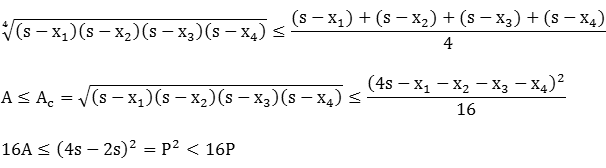since P < 16, Q.E.D.

It is easy to see that there are infinitely-many equable non-cyclic Heronian quadrilaterals (ENCHQs). For example, any rhombus with side length a ≥ 4 and an interior angle equal to Arcsin(4/a) is equable:
A = a2 sin(θ) = 4a = P

θ = Arcsin(4/a)

As the side length increases, the equable rhombus becomes more elongated in order to maintain equal area and perimeter.

Unlike their cyclic counterparts, ENCHQs are not required to have an even perimeter in order to have integer sides and area. Let a,b,c,d be the integer sides of a convex quadrilateral, arranged clockwise with side a horizontally on the bottom, such that the quadrilateral inequality is satisfied. As in the diagram above, let p be the length of the descending diagonal from the vertex joining sides b and c to the vertex joining sides d and a. This diagonal divides the quadrilateral into two triangles; let A1 be the area of the triangle with sides (a,b,p) and A2 the area of the triangle with sides (p,c,d). By equability, we have

A = A1 + A2 = a + b + c + d = P,
or equivalently,
 (16A12 - 16A22)2 - 32(16A12 + 16A22)P2 + 256P4 = 0 
Note that the triangle areas A1 and A2 do not themselves need to be integers, just their sum. Define H1 and H2 as
H1 = 16A12

H2 = 16A22

Then  can be written
 (H1 - H2)2 - 32(H1 + H2)P2 + 256P4 = 0 
From Heron's area formula, we have
H1 = (p + a + b)(-p + a + b)(p - a + b)(p + a - b)

= -p4 + 2(a2 + b2)p2 - (a2 - b2)2,

and similarly,
H2 = -p4 + 2(c2 + d2)p2 - (c2 - d2)2,
so
H1 - H2 = 2(a2 + b2 - c2 - d2)p2 - (a2 - b2)2 + (c2 - d2)2

= 2Wp2 - X

in terms of the following integers:
W = a2 + b2 - c2 - d2

X = (a2 - b2)2 - (c2 - d2)2

In a similar fashion,
H1 + H2 = -2p4 + 2Yp2 - Z
in terms of the following integers:
Y = a2 + b2 + c2 + d2 > 0

Z = (a2 - b2)2 + (c2 - d2)2 > 0

We can thus write  as
(2Wp2 - X)2 - 32(-2p4 + 2Yp2 - Z)P2 + 256P4 = 0,
or
 c2p4 + c1p2 + c0 = 0, 
where the integer coefficients of the quadratic in p2 are
c2 = 4(W2 + 16P2) > 0

c1 = -4(WX + 16YP2)

c0 = X2 + 32ZP2 + 256P4 > 0

By Descartes' Rule of Signs, there can be positive solutions for p2 only when c1 < 0. There are up to two real values of p that satisfy  and do not violate the triangle inequalities or the convexity of the resulting quadrilateral; for example, the (5,5,3,4) quadrilateral with descending diagonals 6.28631 and 5.13017: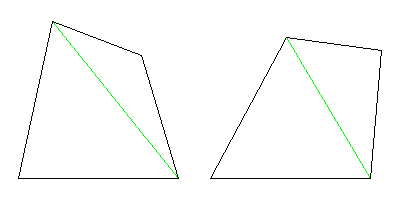### References

[A] Peter, Maximizing the Area of a Quadrilateral, The College Mathematics Journal, Vol. 34, No. 4 (Sept. 2003), pp. 315-316.

[B] Garza-Hume et. al., Areas and Shapes of Planar Irregular Polygons, Forum Geometricorum, 18, p. 25.

[C] Böröczky, K. et. al., The minimum area of a simple polygon with given side lengths, Periodica Mathematica Hungarica, 39, Numbers 1-3 (2000) 33-49.